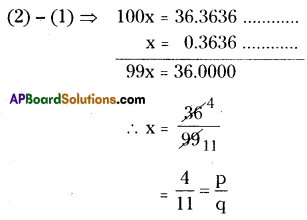# AP Board 8th Class Maths Solutions Chapter 1 Rational Numbers Ex 1.3

AP State Syllabus AP Board 8th Class Maths Solutions Chapter 1 Rational Numbers Ex 1.3 Textbook Questions and Answers.

## AP State Syllabus 8th Class Maths Solutions 1st Lesson Rational Numbers Exercise 1.3Question 1.
Express each of the following decimal in the $$\frac{p}{q}$$ form.
(i) 0.57 (ii) 0.176 (iii) 1.00001 (iv) 25.125
Solution:
(i) 0.57 = $$\frac{57}{100}$$ (∵ two digits are there after the decimal poing)
(ii) 0.176 = $$\frac{176}{1000}$$
(iii) 1.00001 = $$\frac{100001}{100000}$$
(iv) 25.125 = $$\frac{25125}{1000}$$

Question 2.
Express each of the following decimals in the rational form $$\frac{p}{q}$$
(1) $$0 . \overline{9}$$
(ii) $$0 . \overline{57}$$
(iii) $$0 .7 \overline{29}$$
(iv) $$12.2 \overline{8}$$
Solution:
(i) $$0 . \overline{9}$$
Let x = $$0 . \overline{9}$$
⇒ x = 0.999 ………………. (1)
Here periodicity is 1. So, equation (1) should be multiplied both sides with
= 10 × x = 10 × 0.999
10 x = 9.999 ………….. (2)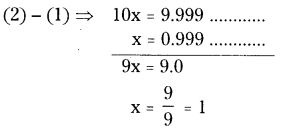$$0 . \overline{9}$$ = 1Second Method:
$$0 . \overline{9}=0+\overline{9}=0+\frac{9}{9}$$
= 0 + 1 = 1

(ii) $$0 . \overline{57}$$
x = $$0 . \overline{57}$$ ⇒ x = 0.5757…………(1)
Here periodicity is 2. So, we should multiply with 100
⇒ 100 × x = 100 x 0.5757 …………..
100 × =57.57 ……………………. (2)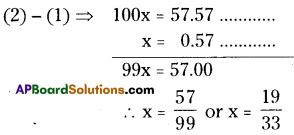(iii) $$0 .7 \overline{29}$$
x = $$0 .7 \overline{29}$$
x = $$0 .7 \overline{29}$$ ⇒ x = 0.7979…………(1)
Here periodicity is 2. So, equation (1) should multiply with 100
⇒ 100 × x = 100 × 0.72929 …………..
100 × = 72.929 …………………… (2)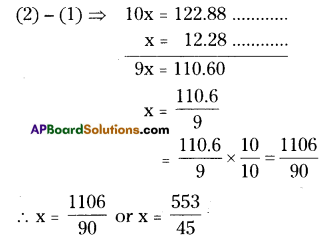(iv) $$12.2 \overline{8}$$
x = (iv) $$12.2 \overline{8}$$
⇒ x = 12.288 ………..(1)
Here periodicity is 1. So, equation (1) should multiply with 10
⇒ 100 × x = 100 × 12.288 …………..
10 x = 122.888 …………………… (2)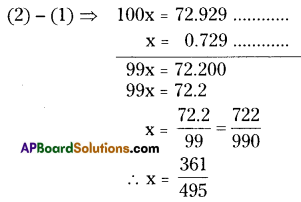Question 3.
Find(x + y) ÷ (x – y) if
(i) x = $$\frac{5}{2}$$, y = $$-\frac{3}{4}$$
(ii) x = $$\frac{1}{4}$$, y = $$\frac{3}{2}$$
Solution:
If x = $$\frac{5}{2}$$, y = $$-\frac{3}{4}$$ then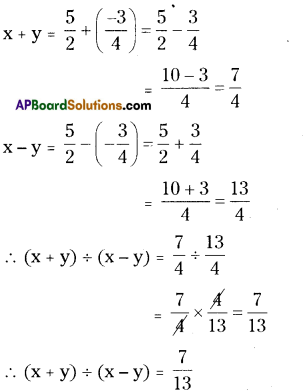ii) x = $$\frac{1}{4}$$, y = $$\frac{3}{2}$$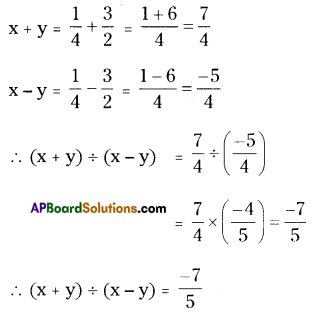Question 4.
Divide the sum of $$-\frac{13}{5}$$ and $$\frac{12}{7}$$ by the product of $$-\frac{13}{7}$$ and $$-\frac{1}{2}$$
Solution:
Sum of $$-\frac{13}{5}$$ and $$\frac{12}{7}$$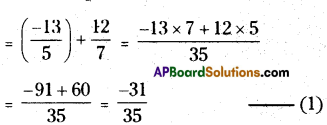the product of $$-\frac{13}{7}$$ and $$-\frac{1}{2}$$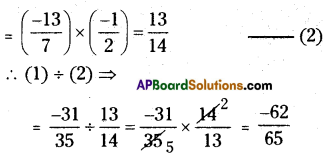Question 5.
If $$\frac{2}{5}$$ of a number exceeds $$\frac{1}{7}$$ of the same number by 36. Find the number.
Solution:
Let the number be ‘x’ say.
$$\frac{2}{5}$$ part of x = $$\frac{2}{5}$$ × x = $$\frac{2x}{5}$$
$$\frac{1}{7}$$ part of x = $$\frac{1}{7}$$ × x = $$\frac{x}{7}$$
∴ According to the sum,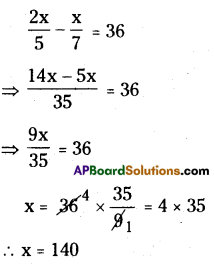Question 6.
Two pieces of lengths 2$$\frac{2}{5}$$ m and 3$$\frac{3}{10}$$ mare cut off from a rope 11 m long. What is the length of the remaining rope?
Soltuion:
The length of the remaining rope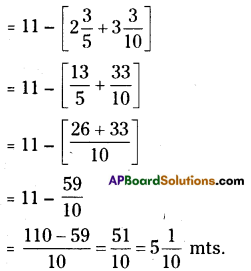∴ The length of remaining rope
= 5$$\frac{1}{10}$$ mts.Question 7.
The cost of 7$$\frac{2}{3}$$ meters of cloth is ₹12$$\frac{3}{4}$$ . Find the cost per metre.
Solution:
The cost of 7$$\frac{2}{3}$$ mts ($$\frac{23}{3}$$ mts ) of cloth
= ₹ $$12 \frac{3}{4}$$ = ₹ $$\frac{51}{4}$$
∴ The cost of 1m cloth
= $$\frac{51}{4} \div \frac{23}{3}=\frac{51}{4} \times \frac{3}{23}=\frac{153}{92}$$ = ₹ 1.66

Question 8.
Find the area of a rectangular park which is 18$$\frac{3}{5}$$m long and 8$$\frac{2}{3}$$ in broad.
Solution:
The length of the rectangular park
= 18$$\frac{3}{5}$$m = $$\frac{93}{5}$$
Its width / breath = 8$$\frac{2}{3}$$ m = $$\frac{26}{3}$$ m
∴ Area of the rectangular park
(A) = l × b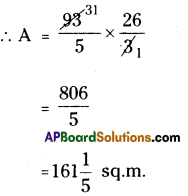Question 9.
What number should $$-\frac{33}{16}$$ be divided by to get $$-\frac{11}{4}$$
Solution:
Let the dividing number be ‘x’ say.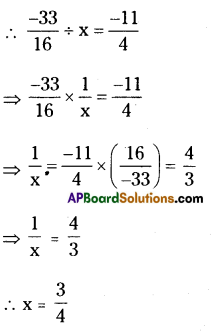Question 10.
If 36 trousers of equal sizes can be stitched with 64 meters of cloth. What is the length of the cloth required for each trouser?
Solution:
36 trousers of equal sizes can he stitched with 64 mts of cloth, then the length of the cloth ¡s required for each trouser
= 64 ÷ 36
= $$\frac{64}{36}=\frac{16}{9}$$ = 1 $$\frac{7}{9}$$Question 11.
When the repeating decimal 0.363636 …. is written in simplest fractional form$$\frac{p}{q}$$ , find the sum p+ q.
Solution:
x = 0.363636………………………….. (1)
Here periodicity is ‘2’. So, equation (1) should be multiplied both sides with 100.
⇒ 100 × x = 100 × 0.363636 …………..
100 x = 36.3636 ………..(2)